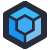We're taking a little summer break this week for our regular articles. Since we're re-using articles, let's start with one about developer efficiency. Why write code when you can automate writing code? Original -- Remy

Several years ago, Dan D’s predecessor, Steve, came to the realization that many of us arrived at one point or another: writing data-access code can get boring and repetitive. To ease the tedium, Steve did what many developers in his position do. He wrote some code to generate a lot of code.

Unfortunately, Steve’s coding skills weren’t all too hot. Worse were his code-writing-code writing skills. In the years since The Great Code Spawn (as it has come to be known), the data-access layer has become an unmaintainable disaster – so much so that, rather than add a new database column, developers have “split” single fields into multiple through bit-shifting and string manipulation.

Following is a single line of code (re-formatted by yours truly) that represents “one of the smaller” insert statements.

```new OdbcCommand(new StringBuffer()
.append(new StringBuffer()
.append(new StringBuffer()
.append(new StringBuffer()
.append(new StringBuffer()
.append(new StringBuffer()
.append(new StringBuffer()
.append(new StringBuffer()
.append(new StringBuffer()
.append(new StringBuffer()
.append(new StringBuffer()
.append(new StringBuffer()
.append(new StringBuffer()
.append(new StringBuffer()
.append(new StringBuffer()
.append(new StringBuffer()
.append(new StringBuffer()
.append(new StringBuffer()
.append(new StringBuffer()
.append(new StringBuffer()
.append("Insert into wc_scanbol values(")
.append(num)
.ToString())
.append(",'")
.ToString())
.append(bol.BolNum)
.ToString())
.append("',")
.ToString())
.append(Integer.parseInt(bol.get_ShipDate().ToString("yyyyMMdd")))
.ToString())
.append(",")
.ToString())
.append(bol.Period)
.ToString())
.append(",'")
.ToString())
.append(bol.Consignee.Replace("'", "''"))
.ToString())
.append("',")
.ToString())
.append(bol.Cases)
.ToString())
.append(",")
.ToString())
.append(bol.Bottles)
.ToString())
.append(",")
.ToString())
.append(num)
.ToString())
.append(",")
.ToString())
.append(bol.CustID)
.ToString())
.append(",'")
.ToString())
.append(bol.State)
.ToString())
.append("','')")
.ToString(), this.con)
.ExecuteNonQuery();```[Advertisement] ProGet’s got you covered with security and access controls on your NuGet feeds. Learn more.